# Pumps

The tank is filled with two pumps in 16 minutes. The first pump is filled in 30 minutes earlier than two one. How many minutes is filled with the first pump?

t1 =  22.9 min

### Step-by-step explanation: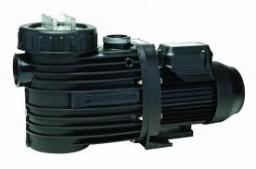Did you find an error or inaccuracy? Feel free to write us. Thank you!Tips to related online calculators
Looking for help with calculating roots of a quadratic equation?
Check out our ratio calculator.
Do you have a linear equation or system of equations and looking for its solution? Or do you have a quadratic equation?

## Related math problems and questions:

• Tank 9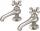The tank with volume V is filled with one pump for three hours and by the second pump for 5 hours. When both pumps run simultaneously, calculate: a) how much of the total volume of the tank is filled in one hour b) for how long is the tank full
• Three pumps together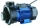One pump fills the tank in 1.5 hours, the second in 2 hours, and the third in 3 hours 20 minutes. How many minutes will the tank fill with three pumps if they work simultaneously?
• Five pumpsThe water tank is filled with two pumps in 48 minutes. How long would it take to fill it with 5 same pumps?
• Three pumps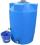The water tank is emptied with the first pump in 6 hours, with the second pump in 2 hours. In order to be emptied in 1 hour, all three pumps had to be started at once. How long will take tank be emptied by only the third pump?
• Pumps A and BPump A fill the tank for 12 minutes, pump B for 24 minutes. How long will take to fill the tank if he is only three minutes works A and then both pumps A and B?
• Pumps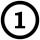First pump flows 16 liters per second into the basin by the second pump 75% of the first and by third pump half more than the second. How long will take fill basin by all three pumps simultaneously volume 15 m3 (cubic meters)?
• Forestry workersIn the forest is employed 56 laborers planting trees in nurseries. For 8 hour work day would end job in 37 days. After 16 days, 9 laborers go forth? How many days are needed to complete planting trees in nurseries by others if they work 10 hours a day?
• Two pumps togetherThe first pump will fill the tank itself in 3 hours and the second one in 6 hours. How many hours will the tank be full if both pumps are worked at the same time?
• Two pumps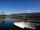The first pump fills the tank in 24 hours second pump in another 40 hours. For how long will it take to fill the tank if it operates both pumps at the same time?
• PumpingThe tank is filled with a larger pump in 12 hours and with scond pump in 15 hours. For how long does the tank fill when both pumps are switched on at the same time?
• 2 pipes2 pipes can fill a tank in 35 minutes. The larger pipe alone can fill the tank in 24 minutes less time than the smaller pipe. How long does each pipie take to fill the tank alone?
• In and out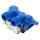The empty tank is filled in 12 minutes and empty in 16 minutes. How long does it take to fill if we forgot to close the drain? If the tank has 1000 l.
• James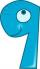James paints a fence. If every day instead of 14 planks painted 16 would be done on one day earlier. How many of planks has a fence altogether?
• Pumps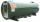6 pump fills the tank for 3 and a half days. How long will fill the tank 7 equally powerful pumps?
• Two pipes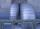One pipe fill one-fifth volume 20 minutes before by second one. The two pipes together will fill the tank in two hours. How long is will fill tank each pipe separately?
• Three pumpsWe are filling the pool. The first pump would be filled in 12 hours, the second pump in 15 hours. If all three pumps were running at the same time, it would fill the pool for 4 hours. How long would the pool fill only with the third pump?
• Two pumpsPump A takes twice as long to fill a tank as B. Together they take 2 hours to fill the tank. How long does each one take to fill the tank by itself?